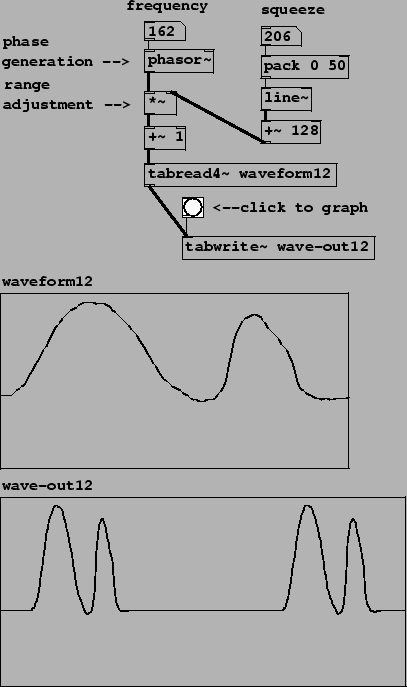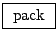Next: Using a wavetable as Up: Examples Previous: Wavetable oscillator   Contents   Index

## Wavetable lookup in general

The tabosc4~ class, while handy and efficient, is somewhat specialized and for many of the applications described in this chapter we need something more general. Example B03.tabread4.pd (Figure 2.13) demonstrates the timbre stretching technique discussed in Section 2.4. This is a simple example of a situation where tabosc4~ would not have sufficed. There are new classes introduced here:: wavetable lookup. As in tabosc4~ the table is read using 4-point interpolation. But whereas tabosc4~ takes a frequency as input and automatically reads the waveform in a repeating pattern, the simpler tabread4~ expects the table lookup index as input. If you want to use it to do something repetitious, as in this example, the input itself has to be a repeating waveform. Like tabosc4~ (and all the other table reading and writing objects), you can send messages to select which table to use.: record an audio signal into a wavetable. In this example the tabwrite~ is used to display the output (although later on it will be used for all sorts of other things.) Whenever it receives a bang" message from the pushbutton icon above it, tabwrite~ begins writing successive samples of its input to the named table.

Example B03.tabread4.pd shows how to combine a phasor~ and a tabread4~ object to make a wavetable oscillator. The phasor~'s output ranges from 0 to 1 in value. In this case the input wavetable, named waveform12", is 131 elements long. The domain for the tabread4~ object is thus from 1 to 129. To adjust the range of the phasor~ accordingly, we multiply it by the length of the domain (128) so that it reaches between 0 and 128, and then add 1, effectively sliding the interval to the right by one point. This rescaling is accomplished by the *~ and +~ objects between the phasor~ and the tabread4~.

With only these four boxes we would have essentially reinvented the tabosc4~ class. In this example, however, the multiplication is not by a constant 128 but by a variable amount controlled by the squeeze" parameter. The function of the four boxes at the right hand side of the patch is to supply the *~ object with values to scale the phasor~ by. This makes use of one more new object class:: compose a list of two or more elements. The creation arguments establish the number of arguments, their types (usually numbers) and their initial values. The inlets (there will be as many as you specified creation arguments) update the values of the message arguments, and, if the leftmost inlet is changed (or just triggered with a bang" message), the message is output.

In this patch the arguments are initially 0 and 50, but the number box will update the value of the first argument, so that, as pictured, the most recent message to leave the pack object was 206 50". The effect of this on the line~ object below is to ramp to 206 in 50 milliseconds; in general the output of the line~ object is an audio signal that smoothly follows the sporadically changing values of the number box labeled squeeze".

Finally, 128 is added to the squeeze" value; if squeeze" takes non-negative values (as the number box in this patch enforces), the range-setting multiplier ranges the phasor by 128 or more. If the value is greater than 128, the effect is that the rescaled phasor spends some fraction of its cycle stuck at the end of the wavetable (which clips its input to 129). The result is that the waveform is scanned over some fraction of the cycle. As shown, the waveform is squeezed into 128/(128+206) of the cycle, so the spectrum is stretched by a factor of about 1/2.

For simplicity, this patch is subtly different from the example of Section 2.4 in that the waveforms are squeezed toward the beginning of each cycle and not toward the middle. This has the effect of slightly changing the phase of the various partials of the waveform as it is stretched and squeezed; if the squeezing factor changes quickly, the corresponding phase drift will sound like a slight wavering in pitch. This can be avoided by using a slightly more complicated arrangement: subtract 1/2 from the phasor~, multiply it by 128 or more, and then add 65 instead of one.Next: Using a wavetable as Up: Examples Previous: Wavetable oscillator   Contents   Index
Miller Puckette 2006-12-30